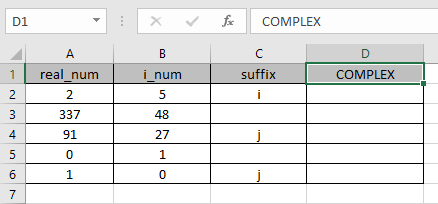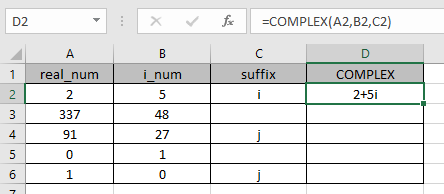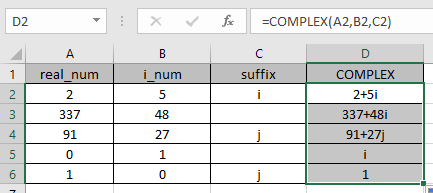# How to use the COMPLEX Function in Excel

In this article, we will learn about how to use the COMPLEX function in Excel.
COMPLEX function in excel derived for mathematical operation have imaginary coefficients. In mathematics we call it the coefficient of i or j.
i = (-1)1/2
Square root of negative number is not possible, so for calculation purpose, -1is named as imaginary and call be iota (i or j). For calculation of some term like shown below.

A = 2 + (-25)1/2

A = 2 + (-1 * 25)1/2

A = 2 + (-1 * 5 * 5)1/2

A = 2 + 5 * (-1)1/2

X + iY = 2 + 5i

This here equation is Complex equation having 2 different parts called real part & complex or imaginary part

The coefficient of iota (i) which is 5 is called as imaginary part and the other part 2 is called the real part.
The COMPLEX function takes the coefficient of real part and imaginary part and returns the numbers in the complex format

Syntax:

=COMPLEX (real_num, i_num, [suffix])

real_num : number as a real part of the complex format
i_num : number as an imaginary part of the complex format
[suffix] : Use i or j as preference. default is set to i (iota)

Let’s understand this function using it in an example.Here we have values where we need to combine them in complex format.

Use the formula:

=COMPLEX (A2, B2, C2)As you can see the values having real_num = 2 & imaginary part = 5. The formula returned the values in the complex function format.

Now copy the formula to the other remaining cells using Ctrl + D shortcut key.As you can see the formula giving results just fine.

Blank cells in the suffix column, so the formula returns the suffix as default i (iota).

 real_num i_num result 0 1 = 0 + 1i = i 1 0 = 1 + 0i = 1

Notes:

1. The function returns the #VALUE! error if Suffix is not in lowercase for i or j.
2. The function returns #VALUE error if the real_num & i_num arguments are non-numeric
3. In absence of suffix argument, the function returns the default i (iota).

Hope you understood how to use COMPLEX function and referring cell in Excel. Explore more articles on Excel function here. Please feel free to state your query or feedback for the above article.

Related Articles

How to use the IMEXP Function in Excel

How to use the IMSIN Function in Excel

How to use the IMSUM Function in Excel

How to use the IMSUB Function in Excel

How to use the SQRT Function in Excel

How to use the IMARGUMENT Function in Excel

How to use the IMCOS Function in Excel

Popular Articles:

50 Excel Shortcuts to Increase Your Productivity

How to use the VLOOKUP Function in Excel

How to use the COUNTIF in Excel 2016

How to use the SUMIF Function in Excel

Edit a dropdown list

If with conditional formatting

If with wildcards

Vlookup by date

Terms and Conditions of use

The applications/code on this site are distributed as is and without warranties or liability. In no event shall the owner of the copyrights, or the authors of the applications/code be liable for any loss of profit, any problems or any damage resulting from the use or evaluation of the applications/code.